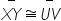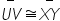Mathematics
Easy

Question

# Name the property illustrated by the statement.If, then## Transitive Property of EqualityReflexive Property of EqualitySymmetric Property of EqualityDistributive PropertyHint:

## The correct answer is: Symmetric Property of Equality

### Here we have to find the reason of (UV) ̅ ≅ (XY) ̅.Firstly , we have given that (XY) ̅ ≅ (UV) ̅ .And also this is given that (UV) ̅ ≅ (XY) ̅ So according to reflexive property if X = Y then it Y = X. And here also (UV) ̅ ≅ (XY) ̅ then (XY) ̅ ≅ (UV) ̅ .Therefore , it follow the property of reflexive .The correct answer is Reflexive property of equality .

In this question, (UV) ̅ ≅ (XY) ̅ and then (XY) ̅ ≅ (UV) ̅ because of the property of reflexive. Reflexive property of equality is say that if X = Y then Y = X also.

### Related Questions to study#### With Turito Foundation.#### Get an Expert Advice From Turito.